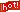4月前 北大袁萌超实数及其应用

当前，全国高校拒绝超实数，清华、北大、浙大亦不例外。为什么？

接受超实数，现有微积分教学大纲就要“翻天”。

为此，我们推荐本文附件文章“Hyperreals and Their Applications”，）供给他们参考。

该文的92篇引用文献全部都是关于超实数的最新研究成果（Pdf文件）

敬请关注。

Hyperreals and Their Applications

Sylvia Wenmackers

Formal Epistemology Project Faculty of Philosophy University of Groningen Oude Boteringestraat 52 9712 GL Groningen The Netherlands E-mail: s.wenmackers@rug.nl URL: http://www.sylviawenmackers.be/

Overview

Hyperreal numbers are an extension of the real numbers, which contain innitesimals and innite numbers. The set of hyperreal numbers is denoted by R or R; in these notes, I opt for the former notation, as it allows us to read the -symbol as the prex ‘hyper-’. Just like standard analysis (or calculus) is the theory of the real numbers, non-standard analysis (NSA) is the theory of the hyperreal numbers. NSA was developed by Robinson in the 1960’s and can be regarded as giving rigorous foundations for intuitions about innitesimals that go back to Leibniz (at least). This document is prepared as a handout for two tutorial sessions on “Hyperreals and their applications”, presented at the Formal Epistemology Workshop 2012 (May 29–June 2) in Munich. It is set up as an annotated bibliography about hyperreals. It does not aim to be exhaustive or to be formally precise; instead, its goal is to direct the reader to relevant sources in the literature on this fascinating topic. The document consists of two parts: sections 1–3 introduce NSA from dierent perspectives and sections 4–9 discuss applications, with an emphasis on topics that may be of interest to formal epistemologists and to philosophers of mathematics or science.

1

Part 1: Introducing the hyperreals

Abstract

NSA can be introduced in multiple ways. Instead of choosing one option, these notes include three introductions. Section 1 is best-suited for those who are familiar with logic, or who want to get a avor of model theory. Section 2 focuses on some common ingredients of various axiomatic approaches to NSA, including the star-map and the Transfer principle. Section 3 explains the ultrapower construction of the hyperreals; it also includes an explanation of the notion of a free ultralter.

1 Existence proofs of non-standard models

1.1 Non-standard models of arithmetic

The second-order axioms for arithmetic are categoric: all models are isomorphic to the intended model N,0,+1. Dedekind was the rst to prove this [Dedekind, 1888b]; his ‘rules’ for arithmetic were turned into axioms a year later by Peano, giving rise to what we now call “Peano Arithmetic” (PA) [Peano, 1889]. The rst-order axioms for arithmetic are non-categoric: there exist nonstandard modelsN,0,+1that are not isomorphic toN,0,+1. Skolem proved this based on the Compactness property of rst-order logic (FOL) [Skolem, 1934]. With the L¨owenheim-Skolem theorem, it can be proven that there exist models of any cardinality. N contains nite numbers as well as innite numbers. We now call N a set of hypernatural numbers. For a discussion of the order-type of countable non-standard models of arithmetic, see e.g. [Boolos et al., 2007, Chapter 25, p. 302–318] and [McGee, 2002]. More advanced topics can be found in this book: [Kossak and Schmerl, 2006].

1.2 Non-standard models of real closed elds

The second-order axioms for the ordered eld of real numbers are categoric: all models are isomorphic to the intended model R,+,×,. A “real closed eld” (RCF) is a eld which has the same rst-order properties as R. Robinson realized that Skolem’s existence proof of nonstandard models of arithmetic could be applied RCFs too. He thereby founded the eld of non-standard analysis (NSA) [Robinson, 1966]. The axioms for RCFs (always in FOL) are non-categoric: there exist non-standard models R,+,×, that are not isomorphic to R,+,×,. With the L¨owenheim-Skolem theorem it can be proven that there exist models of any cardinality; in particular, there are countable models (cf. Skolem ‘paradox’ [Skolem, 1922]).

（全文请见“无穷小微积分”）

2网站